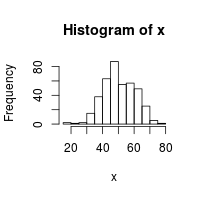## Problem

You want to generate random numbers.

## Solution

For uniformly distributed (flat) random numbers, use `runif()`. By default, its range is from 0 to 1.

``````runif(1)
#>  0.09006613

# Get a vector of 4 numbers
runif(4)
#>  0.6972299 0.9505426 0.8297167 0.9779939

# Get a vector of 3 numbers from 0 to 100
runif(3, min=0, max=100)
#>  83.702278  3.062253  5.388360

# Get 3 integers from 0 to 100
# Use max=101 because it will never actually equal 101
floor(runif(3, min=0, max=101))
#>  11 67  1

# This will do the same thing
sample(1:100, 3, replace=TRUE)
#>   8 63 64

# To generate integers WITHOUT replacement:
sample(1:100, 3, replace=FALSE)
#>  76 25 52
``````

To generate numbers from a normal distribution, use `rnorm()`. By default the mean is 0 and the standard deviation is 1.

``````rnorm(4)
#>  -2.3308287 -0.9073857 -0.7638332 -0.2193786

# Use a different mean and standard deviation
rnorm(4, mean=50, sd=10)
#>  59.20927 40.12440 44.58840 41.97056

# To check that the distribution looks right, make a histogram of the numbers
x <- rnorm(400, mean=50, sd=10)
hist(x)
``````If you want to your sequences of random numbers to be repeatable, see ../Generating repeatable sequences of random numbers.